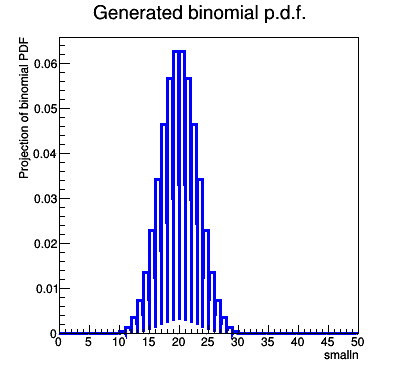# Converting RooRealVar into integer to generate binomial pdf

Dear Rooters,

I’m trying to generate a binomial pdf which I can then fit to my measured data. Problem is RooFit doesn’t seem allow the user to declare an integer variable that is to be used to generate the pdf.

Is there a work around? see sample code and output below:

many thanks in advance!

code

``````#ifndef __CINT__
#include "RooGlobalFunc.h"
#endif
#include "RooRealVar.h"
#include "RooDataSet.h"
#include "TMath.h"
#include "TCanvas.h"
#include "RooPlot.h"
#include "TAxis.h"
using namespace RooFit ;

void FitGeneratedBinomialModelToData()
{
// Declare variables
RooRealVar p("p","p",0.5,0,1) ;
RooRealVar smalln("smalln","smalln",1,0,50) ;
RooRealVar bigN("bigN","bigN",40,39,41) ;
// build pdf
RooGenericPdf binomialpdf("binomial","binomial PDF","pow(p,smalln)*pow((1-p),(bigN-smalln))*TMath::Factorial(bigN)/(TMath::Factorial(smalln)*TMath::Factorial(bigN-smalln))",RooArgSet(smalln,p,bigN)) ;

// Construct plot frame in 'm'
RooPlot* xframe = smalln.frame(Title("Generated binomial p.d.f.")) ;
// Plot
binomialpdf.plotOn(xframe) ;

// Draw all frames on a canvas
TCanvas* c = new TCanvas("binomialPdf","binomialPdf",400,400) ;
gPad->SetLeftMargin(0.15) ; xframe->GetYaxis()->SetTitleOffset(1.6) ; xframe->Draw() ;
}``````

The output (below) is clearly wrong and occurs because RooFit computes the Pdf by stepping "smalln in steps of 0.5 rather than with integer stepsizeDuplication of post